# Unknown mixed number

Find the number that is smaller than 5 5/12 by as much as 2 2/13 is smaller than 6 1/6

x =  1.4038

### Step-by-step explanation: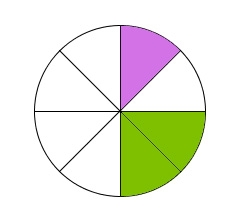Did you find an error or inaccuracy? Feel free to write us. Thank you!Tips to related online calculators
Need help to calculate sum, simplify or multiply fractions? Try our fraction calculator.
Do you have a linear equation or system of equations and looking for its solution? Or do you have a quadratic equation?

## Related math problems and questions:

• Find unknown 2Find unknown denominator: 2/3 -5/? = 1/4
• Unknown numberFind an unknown number of which 1/5 is 40 greater than one-tenth of that number.
• Unknown number 716% of the unknown number is by 21 less than unknown number itself. Determine the natural unknown number.
• The difference 2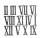The difference between the two numbers is 25. The smaller number is 1/6th of the larger number. What is the value of the smaller number?
• Unknown number 4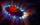I think number. When I divided its square root by its 1/9, I get a number 1. What number am I thinking?
• Two unknown numbersOne number is 2 less than a second number. Twice the second number is 14 less than 5 times the first. Find the two numbers.
• Unknown number 5I think of an unknown number. If we enlarge it five times, then subtract 3, and the result decreases by 75%, we get one greater than the number. What number am I thinking of?
• Unknown number 6Determine the unknown number, which is by 1.5 greater than its fourth.
• Divide 11Divide the product of 4 and 5/8 by 1 1/2. Write your answer as a mixed number.
• Fractions and mixed numerals(a) Convert the following mixed numbers to improper fractions. i. 3 5/8 ii. 7 7/6 (b) Convert the following improper fraction to a mixed number. i. 13/4 ii. 78/5 (c) Simplify these fractions to their lowest terms. i. 36/42 ii. 27/45 2. evaluate the follow
• FractionsThree-quarters of an unknown number is 4/5. What is 5/6 of this unknown number?
• One dice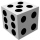Calculate the probability of one dice roll with the numbers 1, 2, 3, 4, 5, 6 on the walls. Write the results in a notebook in the shape of a fraction in the basic form: 2/3. a, The number 1 falls on the cube. b, The number 5 falls on the cube. c, An even
• Unknown number 7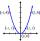Calculate unknown number whose 12th power when divided by the 9th power get a number 27 times greater than the unknown number. Determine the unknown number.
• Unknown numberFind the unknown number equal to a quarter of a fifth of a number, which is by 152 more than an unknown number.
• Unknown number x5% from an unknown number equals the number 26. What is the unknown number?
• One-thirdA one-third of unknown number is equal to five times as great as the difference of the same unknown number and number 28. Determine the unknown number.
• Fraction unknowns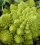Divide of fractions with unknowns: Fraction 1: The quantity x squared plus 6 times x plus 9 over the quantity x minus 1. Fraction 2: the quantity x squared minus 9 over the quantity x squared minus 2 times x plus 1.  Find Fraction 1 over Fraction 2.